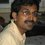# About $x^y=y^x$

Consider the equation $x^y=y^x$ and it is required to find ALL solutions $(x,y)$ for this equation,

It is obvious that all ordered pairs $(x,x)$, i.e., $y=x \ne 0$ is a trivial set of solutions. (Here, I am not sure if $x=y=0$ can be considered as a solution!!)

In addition, the set $x=\alpha^{\frac{1}{\alpha-1}},y=\alpha x$, for some real (not sure if complex works) value of $\alpha \ne 1,0$, would also fit in as solutions.

Are there any solutions which do not fit into the above two categories? Is it possible to prove otherwise?Note by Janardhanan Sivaramakrishnan
5 years, 7 months ago

This discussion board is a place to discuss our Daily Challenges and the math and science related to those challenges. Explanations are more than just a solution — they should explain the steps and thinking strategies that you used to obtain the solution. Comments should further the discussion of math and science.

When posting on Brilliant:

• Use the emojis to react to an explanation, whether you're congratulating a job well done , or just really confused .
• Ask specific questions about the challenge or the steps in somebody's explanation. Well-posed questions can add a lot to the discussion, but posting "I don't understand!" doesn't help anyone.
• Try to contribute something new to the discussion, whether it is an extension, generalization or other idea related to the challenge.

MarkdownAppears as
*italics* or _italics_ italics
**bold** or __bold__ bold
- bulleted- list
• bulleted
• list
1. numbered2. list
1. numbered
2. list
Note: you must add a full line of space before and after lists for them to show up correctly
paragraph 1paragraph 2

paragraph 1

paragraph 2

[example link](https://brilliant.org)example link
> This is a quote
This is a quote
    # I indented these lines
# 4 spaces, and now they show
# up as a code block.

print "hello world"
# I indented these lines
# 4 spaces, and now they show
# up as a code block.

print "hello world"
MathAppears as
Remember to wrap math in $$ ... $$ or $ ... $ to ensure proper formatting.
2 \times 3 $2 \times 3$
2^{34} $2^{34}$
a_{i-1} $a_{i-1}$
\frac{2}{3} $\frac{2}{3}$
\sqrt{2} $\sqrt{2}$
\sum_{i=1}^3 $\sum_{i=1}^3$
\sin \theta $\sin \theta$
\boxed{123} $\boxed{123}$

Sort by:

There are solutions that do not fit. There will be a negative real solution as long as $y=\frac{a}{b}>1$, and there might be 2 negative real solutions when $y=\frac{a}{b}<1$, where $a$ is an even number and $b$ is an odd number. I haven't actually studied this in detail yet.

From what I got so far, numerically, there would be 2 negative real solutions when $y=\frac{a}{b}$, $k_c, $0.752688172043

- 5 years, 7 months ago

I do not even restricting $x,y$ to be real numbers.

Take $\alpha = -1$, we get $x=i=e^{\frac{\pi}{2}i},y=-i=e^{\frac{-\pi}{2}i}$ and

thus $x^y=e^{\frac{\pi}{2}i \times (-i)}=e^{\frac{\pi}{2}}$

and also $y^x=e^{\frac{-\pi}{2}i \times (i)}=e^{\frac{\pi}{2}}$

- 5 years, 7 months ago

Related: soumava's algorithm

- 5 years, 4 months ago

$\Large 2^4=4^2$

- 5 years, 7 months ago

This also fits the pattern. Take $\alpha = 2$, $x=2^{\frac{1}{2-1}}=2,y=2*2=4$.

- 5 years, 7 months ago